Function Repository Resource:

# TensorIndexJuggling

Given a metric, convert between covariant and contravariant components of a tensor

Contributed by: Lars Ulke-Winter
 ResourceFunction["TensorIndexJuggling"][tensor,metric] uses metric to convert tensor, having only covariant components (lower indices), into the corresponding tensor with all contravariant components (upper indices). ResourceFunction["TensorIndexJuggling"][tensor,metric,"From"→"AllContravariant","To"→"AllCovariant"] converts tensor, having only contravariant components, into the corresponding tensor with all covariant components. ResourceFunction["TensorIndexJuggling"][tensor,metric,"From"→spec1,"To"→spec2] transforms between upper and lower indices as indicated by "From" and "To".

## Details and Options

Index juggling is done via the covariant tensor metric gij. To convert contravariant components to covariant, use tigij=tj.
Input tensor can be of any rank and should be a List or a StructuredArray.
The speci can be chosen from among the following: "AllCovariant", "AllContravariant" or {c1,c2,}, where the ci are either of the literals "Con" or "Cov".

## Examples

### Basic Examples (4)

Transform a covariant rank-2 tensor in a cylindrical coordinate system to its contravariant form tijtij:

 In:=Out=Transform a covariant rank-2 tensor in cylindrical coordinates to its mixed form tijtij:

 In:=Out=Convert tijtij for a SymmetrizedArray:

 In:=Out=Attempting the conversion tijtij changes nothing:

 In:=Out=### Scope (17)

#### Index juggling of base vectors in cylindrical frame (3)

 In:=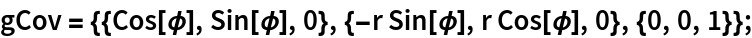Get the contravariant base through raising the index:

 In:=Out=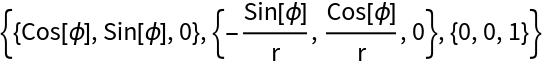Check the orthonormality relationship gi·gj=δij:

 In:=Out=#### Index juggling of the metric tensor in cylindrical frame (4)

 In:=Out=Get the contravariant components of the metric:

 In:=Out=This result is the same as the Wolfram Language built-in function:

 In:=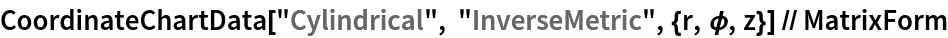Out=In:=Out=#### Contraction of two opposing indices leads to an invariant scalar (6)

 In:=Out=Define a Cartesian rank-2 tensor:

 In:=Out=Contract the indices:

 In:=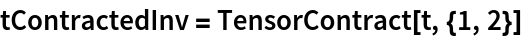Out=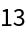Use the resource function TensorCoordinateTransform to transform t to cylindrical coordinates with covariant transformation behavior tij:

 In:=Out=Contraction should not be invariant:

 In:=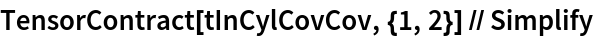Out=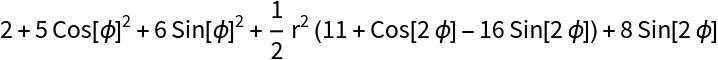But contraction of opposing indices should be:

 In:=Out=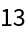#### Contraction of opposing indices in a rank-6 tensor  (4)

Start with a rank-6 tensor in a Cartesian basis and form the contraction tijkijkl:

 In:=Out=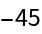Transform to an different affine system with given mapping:

 In:=Contraction of the indices of the same type does not lead to an invariant:

 In:=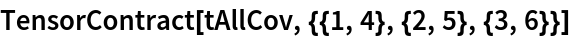Out=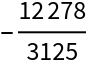Contraction of the right opposing index pairs, after index juggling, leads to the same result as in the original coordinate system:

 In:=Out=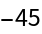### Properties & Relations (1)

If the mapping rule between the two coordinate frames exists, index juggling can also be done using the resource function TensorCoordinateTransform. However, if only the metric of the space is available, then TensorIndexJuggling is recommended:

 In:=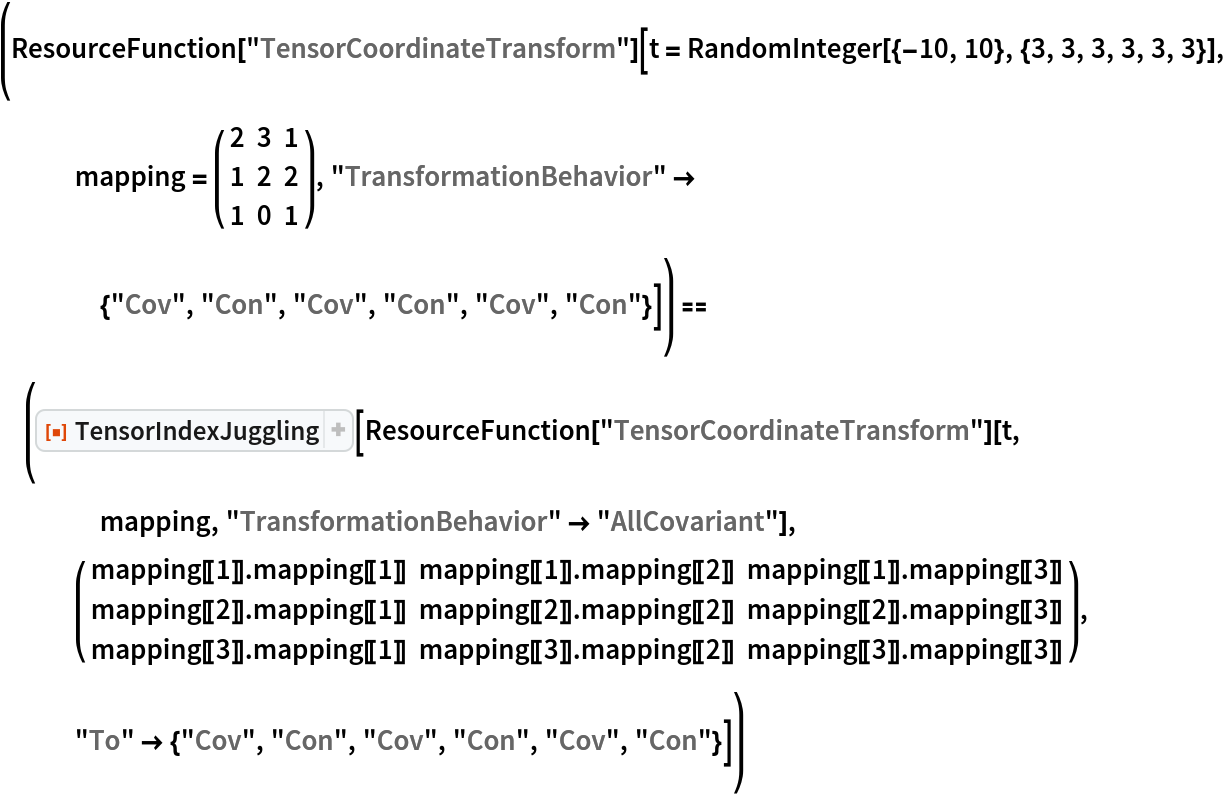Out=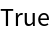Lars Ulke-Winter

## Version History

• 1.0.0 – 03 October 2019